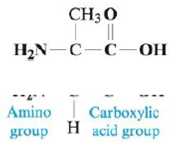# Amino acids are the building blocks for all proteins in our bodies. A structure for the amino acid alanine is All amino acids have at least two functional groups with acidic or basic properties. In alanine, the carboxylic acid group has K a = 4.5 × 10 −3 and the amino group has K b = 7.4 × 10 −5 . Because of the two groups with acidic or basic properties, three different charged ions of alanine are possible when alanine is dissolved in water. Which of these ions would predominate in a solution with [H + ] = 1.0 M ? In a solution with [OH − ] = 1.0 M ?### Chemistry: An Atoms First Approach

2nd Edition
Steven S. Zumdahl + 1 other
Publisher: Cengage Learning
ISBN: 9781305079243

#### Solutions

Chapter
Section### Chemistry: An Atoms First Approach

2nd Edition
Steven S. Zumdahl + 1 other
Publisher: Cengage Learning
ISBN: 9781305079243
Chapter 14, Problem 86AE
Textbook Problem
9 views

## Amino acids are the building blocks for all proteins in our bodies. A structure for the amino acid alanine isAll amino acids have at least two functional groups with acidic or basic properties. In alanine, the carboxylic acid group has Ka = 4.5 × 10−3 and the amino group has Kb = 7.4 × 10−5. Because of the two groups with acidic or basic properties, three different charged ions of alanine are possible when alanine is dissolved in water. Which of these ions would predominate in a solution with [H+] = 1.0 M? In a solution with [OH−] = 1.0 M?

Interpretation Introduction

Interpretation:

The Ka value for the carboxylic acid group and the amino group in alanine is given. The ions that would predominate in a solution with [H+]=1.0M and in a solution with [OH]=1.0M are to be stated.

Concept introduction:

The value of pKa is calculated by the formula, pKa=log(Ka)

The pH of a solution is calculated by the formula, pH=log[H+]

The pOH of a solution is calculated by the formula, pOH=log[OH]

### Explanation of Solution

Explanation

To find the Kb

given value of Ka is 4.5×103 .

The given value of Kb is 7.4×105 .

The value of pKa is calculated by the formula,

pKa=log(Ka)

Substitute the value of Ka in the above expression.

pKa=log(4.5×103)=2.35_

The value of pKb is calculated by the formula,

pKb=log(Kb)

Substitute the value of Kb in the above expression.

pKb=log(7.4×105)=4.13_

To find the pH

The pH of a solution is calculated by the formula,

pH=log[H+]

The value of [H+]=1

### Still sussing out bartleby?

Check out a sample textbook solution.

See a sample solution

#### The Solution to Your Study Problems

Bartleby provides explanations to thousands of textbook problems written by our experts, many with advanced degrees!

Get Started

Find more solutions based on key concepts
What is an ovule?

Biology (MindTap Course List)

Some conditions, such as, are almost entirely nutrition related. a. cancer b. Down syndrome c. iron-deficiency ...

Nutrition: Concepts and Controversies - Standalone book (MindTap Course List)

What are the products of the acid hydrolysis of the local anesthetic lidocaine?

Chemistry for Today: General, Organic, and Biochemistry

(a) A space vehicle is launched vertically upward from the Earths surface with an initial speed of vi that is c...

Physics for Scientists and Engineers, Technology Update (No access codes included)